Orthogonal frame via gram schmidt or eigendecomposition

Functions such as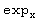: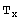M→M require an orthonormal frame with respect to. We use the eigenvectors offind a set of orthonormal vectors. We begin with a numerical treatment. The scalar product considered has index 1.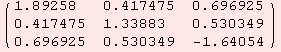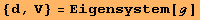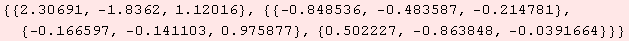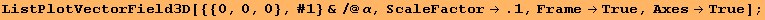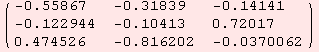An orthonormal vector is in the column of α.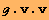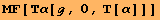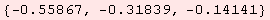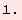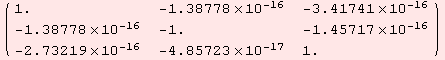The above procedure is carried out byAn alternative way to orthonormalize vectors is via gram schmidt. However, a basis of vectors of length ≠0 is required.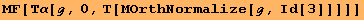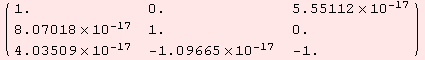Even in low dimensions, expressions get quite lengthy when solving the problem algebraically. As an example, a metric for a coordinate environment for the sphere.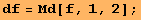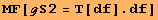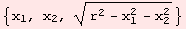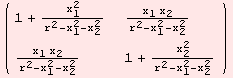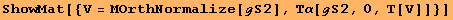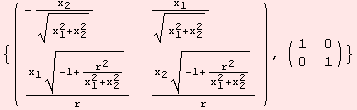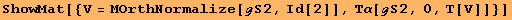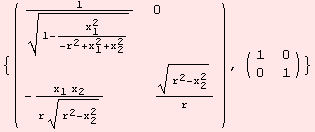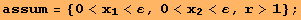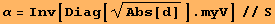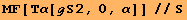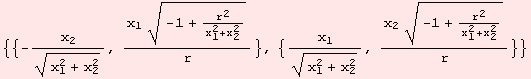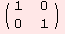Just for fun, we plot f(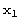,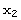) with emphasis on real and imaginary part.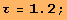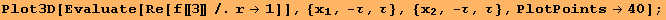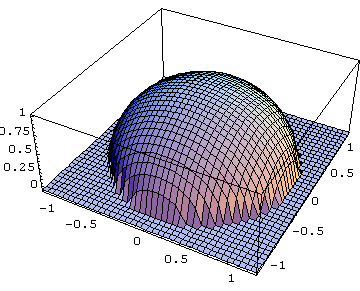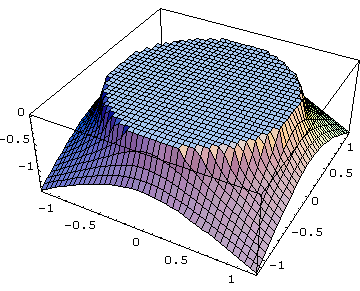The two parts attached are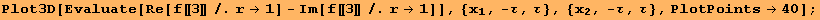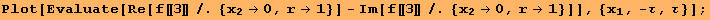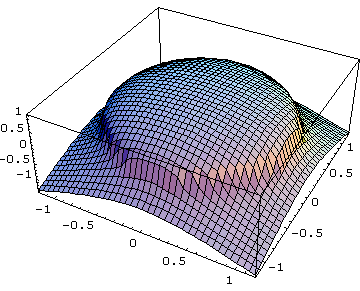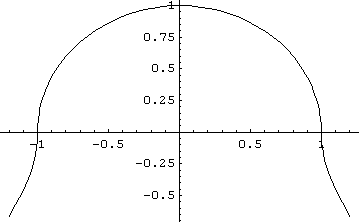Created by Mathematica  (December 22, 2006)